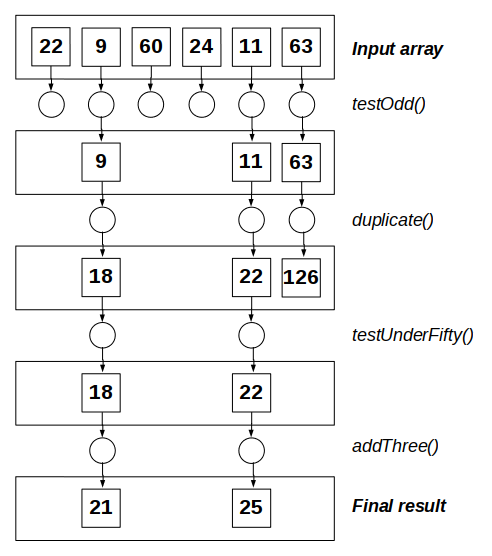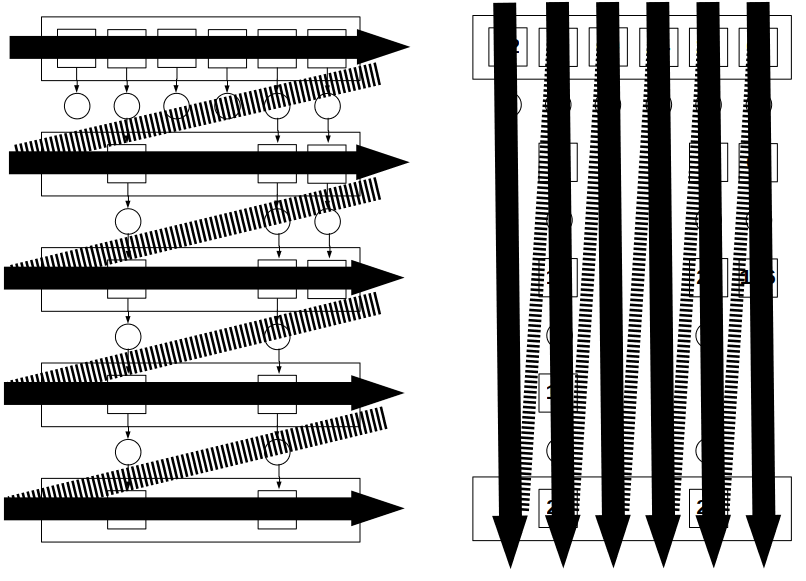Back

# Forever Functional: Maximize JavaScript's performance with transducersDeclarative methods such as map(), filter(), and reduce() have clarity advantages, but in some cases may incur in performance costs. This article will show you how to use transducers to fully optimize any sequence of operations, with no loss of clarity.Get to the root cause of every issue
Observe user actions for quicker issue troubleshooting with OpenReplay.

Discover how at OpenReplay.com.

Working declaratively, the use of `map()`, `filter()` and `reduce()` methods is encouraged. However, when dealing with large arrays and applying a sequence of such operations, many intermediate arrays are created, processed, and finally discarded, all of which cause delays. Sure, for small arrays this will have little impact, but if you have to deal with larger amounts of data (maybe a data stream or the result of a large database query) you’ll have to optimize the process. Fortunately, there’s a clean way to achieve this: using transducers.

For trivia buffs: “transduce” is a word of Latin origin, meaning transform, change, convert, or transport. The term is commonly used in many fields, including biology, electronics, machine learning, and more.

I wrote about this in my Mastering Functional JavaScript Programming (3rd edition) book; let’s recap the main concepts here. We’ll be using TypeScript (not plain JavaScript) and that will allow us to do some work with generic types and higher order functions.

## Getting started

Let’s begin by having some functions and data to work with. I’ll use the examples from the book; they are nonsensical, but that’s OK since we want to focus on the solution and not on any particular case.

``````const testOdd = (x: number): boolean => x % 2 === 1;
const duplicate = (x: number): number => x + x;
const testUnderFifty = (x: number): boolean => x < 50;
const addThree = (x: number): number => x + 3;``````

We will apply these functions in sequence to an array: we’ll filter out the even values, keeping just the odd ones, double the resulting numbers, keep only those under 50, and end by adding three to the results.

``````const myArray = [22, 9, 60, 24, 11, 63];

const a0 = myArray
.filter(testOdd)
.map(duplicate)
.filter(testUnderFifty)

console.log(a0);
// Output: [ 21, 25 ]``````

This is how things work:We started with the original array and then:

1. filtering that array with `testOdd()` produced a second array
2. applying the `duplicate()` map to the second array created a third one
3. filtering the third array with `testUnderFifty()` produced a fourth array, and
4. applying the `addThree()` map to it generated the final result, a fifth array.

With such a small array, you won’t even notice performance issues, but with a larger array, all those array creations can really require a lot of time. We have to think of a better way of proceeding.

## A better approach

How can we manage to optimize this sequence of steps? In essence, we are applying all transformations one at a time; in pseudocode, it would be something like the following algorithm:

``````for each transformation to be applied:
for each element in the input list:
apply the transformation to the element``````

A better approach would be taking elements one by one and applying all transformations in sequence to them, which would directly produce the final result requiring no intermediate arrays. The pseudocode for this is as follows: we are swapping the two loops.

``````for each element in the input list:
for each transformation to be applied:
apply the transformation to the element``````

We have a solution — but how can we implement it? Let’s get to that.

## Implementing the new approach

The question pops up: how can we do all this in a single step? An important concept (check this previous article for more on that) is that you can emulate `map()` and `filter()` by using `reduce()` creatively. The trick is what function to use with our `reduce()` call; everything hinges on that. We’ll change the order of evaluation as shown in the image below but still get the same results in a shorter time.We will use `reduce()` to implement our optimized single-step procedure. We will compose all the transformation functions together; each one will take care of calling the next in sequence. Let’s see the JavaScript code —not really obvious at first glance!— and then explain how it works.

``````const mapTR =
<V, W>(fn: (x: V) => W) =>
<A>(reducer: (am: A, wm: W) => A) =>
(accum: A, value: V): A =>
reducer(accum, fn(value));

const filterTR =
<V>(fn: (x: V) => boolean) =>
<A>(reducer: (af: A, wf: V) => A) =>
(accum: A, value: V): A =>
fn(value) ? reducer(accum, value) : accum;``````

We have created a pair of transducers, higher-order functions that will get a reducing function as input and produce a new reducing function as output. In all cases, `reducer()` is the next transformation in the sequence.

When mapping, we assume a transformation `fn()` function that gets a value of type `V` as input and produces a result of type `W`. The generic reducer takes an accumulator of type `A` and a value of type `W` and produces a new accumulator, also of type `A`. We process the input array of type `V`, use `fn()` to get a value of type `W`, and then call `reducer()` to further process the current result and update the `accum` accumulator.

For filtering, the logic is very similar, but the last step differs. We use `fn()` to decide whether a value must be kept or filtered out. Only in the first case do we use `reducer()` to keep processing the value and updating `accum`; if the test fails, we don’t change the value of the `accum` accumulator.

How do we use these transducers? Let’s see this.

## Using transducers

We will solve our optimization problem by writing code as follows. First, we convert our original functions into transducers.

``````const testOddR = filterTR(testOdd);
const duplicateR = mapTR(duplicate);
const testUnderFiftyR = filterTR(testUnderFifty);

Our transformed functions will calculate their result and call a reducer to further deal with that. We’ll need a final special function, `addToArray()`, to finish the sequence of transformations by actually creating the output array.

``````const addToArray = <V>(a: V[], v: V): V[] => {
a.push(v);
return a;
};``````

So now the solution is direct — though longish!

``````const a1 = myArray.reduce(
[]
);

console.log(a1);
// Output: [ 21, 25 ], again``````

How does all this work?

• `reduce()` is used, with an empty array (which will become the final result) as accumulator
• `testOddR()` does its test and, if odd, passes it to the following function
• `duplicateR()` duplicates the value it received and passes it to the next
• `testUnderFiftyR()` tests the value, and if under 50, passes it to the next
• `addThreeR()` adds 3 to whatever it received, and passes the value to the last function, and
• `addToArray()` just pushes its input to the accumulator array

This works, and in a single pass! But it’s not generic enough; let’s keep on working!

## Generalizing

We can have many `map()` and `filter()` calls, but the code we wrote will always end with an array — what if we wanted to do a final `reduce()`? It would require a new pass over the array, and we wanted to do just ONE pass, no more.

There are two things we need to change: we may not want to use `addToArray()` as the final function in our transducing series, and we may have a different starting value other than `[]`. For instance, if we wanted to find the sum of all the numbers, we could write:

``````const sum = (acc: number, value: number): number => acc + value;

const a2 = myArray.reduce(
0
);

console.log(a2);
// Output: 46``````

Note the two changes: we are passing `sum` and `0` instead of `addToArray` and `[]`, and now we have optimized the sequence of `map()`, `filter()`, and `reduce()` calls to process the input array just once, and directly generate the desired output, without any intermediate arrays; a true gain!

## Summary

Using functional programming doesn’t imply, in any way, that lower performance is to be expected and accepted. In this article, we’ve seen how to transform any sequence of mapping, filtering, and reducing calls into a single `reduce()` pass that will require no intermediate arrays and won’t require any extra time.

We may yet write this in a clearer way by using function composition and writing a `transduce()` higher-order function — but that will be for a future article in this series!

### Understand every bug

Uncover frustrations, understand bugs and fix slowdowns like never before with OpenReplay — the open-source session replay tool for developers. Self-host it in minutes, and have complete control over your customer data. Check our GitHub repo and join the thousands of developers in our community.